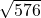Find the radius of a circle whose area is 576π m²

Question

Find the radius of a circle whose area is 576π m²

in progress 0
5 months 2021-08-19T05:01:02+00:00 1 Answers 3 views 0

r = 24 m

Step-by-step explanation:

The area (A) of a circle is calculated as

A = πr² ( r is the radius )

Here A = 576π , then

πr² = 576π ( divide both sides by π )

r² = 576 ( take the square root of both sides )

r == 24